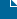## Project Euler #2

In Golf, Project Euler, Ruby on May 11, 2009 by Matt Grande

Here we go, Project Euler #2!

Each new term in the Fibonacci sequence is generated by adding the previous two terms. By starting with 1 and 2, the first 10 terms will be:

1, 2, 3, 5, 8, 13, 21, 34, 55, 89, …

Find the sum of all the even-valued terms in the sequence which do not exceed four million.

```one_back, two_back, current_fib, total = 1, 1, 0, 0</code>

while current_fib &lt; 4_000_000 do
two_back = one_back
one_back = current_fib
current_fib = one_back + two_back
total += current_fib if current_fib % 2 == 0
end

puts total
```

And the golf…

# Score: 69
a,b,c,t=1,0,0,0;while(c<4000000):b=a;a=c;c=a+b;t+=c if c%2==0;end;p t [/sourcecode] EDIT! I knew there was a way to do it without that stupid current_fib variable, but I couldn't get it to work at first. Then, as soon as I post, I realised what I was doing wrong. [sourcecode language="ruby"] one_back, two_back, total = 1, 1, 0 while one_back < 4_000_000 do total += one_back if one_back % 2 == 0 current = one_back one_back += two_back two_back = current end puts total [/sourcecode] And, even better, it improved my golf score! [sourcecode language="ruby"] # Score: 63 a,b,t=1,1,0;while a<4000000:t+=a if a%2==0;c=a;a+=b;b=c;end;p t [/sourcecode]